﻿ Asymptotic Property of Mediant for Lagrange’s Mean Value TheoremPublications are Open
Access in this journal
Article Versions
Export Article
• Normal Style
• MLA Style
• APA Style
• Chicago Style
Research Article
Open Access Peer-reviewed

### Asymptotic Property of Mediant for Lagrange’s Mean Value Theorem

Jianbing Cao
Journal of Mathematical Sciences and Applications. 2017, 5(1), 27-29. DOI: 10.12691/jmsa-5-1-5
Published online: August 30, 2017

### Abstract

In this paper, we mainly discuss the asymptotic property of mediant for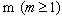order Lagrange’s mean value theorem, and obtain the general results for this problem: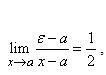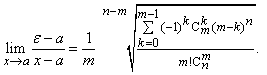### 1. Introduction

Differential mean value theorem is an important theorem in advanced mathematics, over the years, there are many papers concerning the intermediate point of differential mean value theorem, and many related results have been obtained, such as the Lagrange mean value theorem in the literature, the authors studied the second-order, third-order and fourth-order gradual properties of middle value theorem in some cases, For example, for the fourth order mean value theorem, the authors got the following conclusions:In the literature 7, the authors studied the five order situation, and then further generalized the result of the above. Inspired from some research in the literature, and the results given in the similarity, we should naturally consider this question for any order of mean value theorem. In this article, we used the combination mathematics knowledge, we further study the asymptotic property of mediant for any order’s mean value theorem, and obtain the general results for this problem, as a result, we generalize the corresponding results of other authors.

### 2. Some Lemms

In order to prove our main results, we first need the following two lemms.

Lemma 2.1. ( 4) Let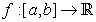be continuous onand has a derivative of orderat the interior points of the interval, then there exists a point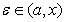such that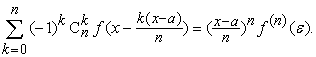(2.1)

Lemma 2.2. ( 2) For any, we have:Corollary 2.3. For any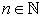, we have: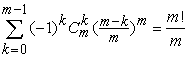(2.2)(2.3)

Proof. We can obtain these two inequalities by simple computation, here we omit the details.

### 3. Main Results

In this section, we will prove our main results in this paper.

Theorem 3.1. Letbe continuous on interval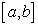and has a derivative of orderat the interior points of the interval. If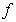has a derivative of order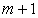at the pointand, then for any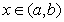, there exists a pointsatisfies (2.1) andProof. For anysince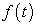is continuous onand has a derivative of order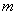at the interior points of the interval, then from (1.1):(3.1)

Now, construct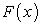such that:(3.2)

where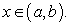Using (3.1), we can obtainNow, let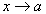, we have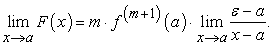(3.3)

On the other hand, applying Hopital’s rule to (3.2):Now, applying Hopital’s rule to for n times:(3.4)

By using Corolly 2.3 and (3.4), we can botian the following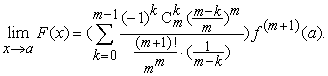(3.5)

Using Corollary 2.3 again, then from (3.5),(3.6)

It follows from (3.6) and (3.6) that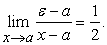This completes the proof.

Theorem 3.2. Letbe continuous on intervaland has a derivative of order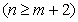at the interior points of the interval. If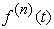is continuous at the pointand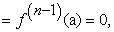then for anythere exists a pointsatisfies (2.1) and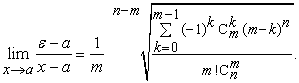Proof. Construct such that(3.7)

Using (3.1), we can obtain(3.8)

Applying Taylor's formula to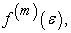we have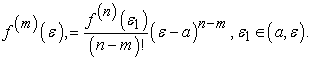(3.9)

Now, applying (3.9) to (3.8),Let, we get(3.10)

On the other hand, applying Hopital’s rule to (3.7) for n times: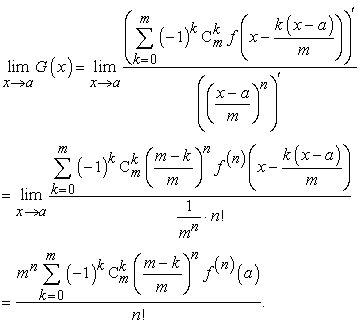(3.11)

It follows from (3.5) and (3.6) that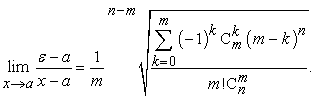This completes the proof.

Remark 3.3. If we putin our Theorem 3.1 and Theorem 3.2, then we can some related results in the literature, thus, we obtain the general results for this problem.

### Acknowledgements

The author is grateful to the referee and editors for their helpful comments and suggestions.

### References

  G. Xu, Asymptotic property of mediant for Lagrange's and Cauchy's theorem of second Order, Journal of Beijing Institute of Clothing, 1990, 201-205. In article View Article  B. Chen, On Several new methods to proof combinatorial identities, Journal of Ningxia University (Natural Science Edition), 2004, 231-233. In article PubMed  Y. Ding, A further research on intermediate point in the second mean value theorem for integrals, Journal of Hefei Teachers College; 2009, 304-306. In article View Article  X. Zhang and W Wang, A class of differential intermediate value theorem and its asymptotic behavior of mediant, Journal of Beijing Institute of Clothing, 2001, 181-185. In article  J. Du, The forms of higher order of Cauchy’s and Lagrange’s mean value theorem, Journal of Huaibei Coal Industry Teachers College, 2001, 211-215. In article  W. Rudin, Principles of mathematical analysis, New York: McGraw-Hill.1976. In article View Article  T. M Apostol, Mathematical analysis, Addison-Wesley Pub. Co.1974. In article View Article  W, Wang, Asymptotic Behaviour of mediant for integral F form Cauchy's mean value theorem, Journal of Beijing Institute of Clothing, 2005, 25-27. In article View Article  Q. Zheng, The asymptotic property of the intermediate value of the first mean value theorem for the integrals and the quadrature formula, College Mathematies, 2004, 6-9. In article PubMedThis work is licensed under a Creative Commons Attribution 4.0 International License. To view a copy of this license, visit http://creativecommons.org/licenses/by/4.0/